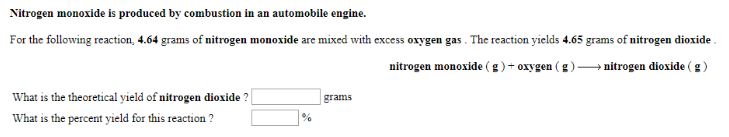# Problem: Nitrogen monoxide is produced by combustion in an automobile engine.For the following reaction, 4.64 grams of nitrogen monoxide are mixed with excess oxygen gas. The reaction yields 4.65 grams of nitrogen dioxide.nitrogen monoxide (g) + oxygen (g) → nitrogen dioxide (g) What is the theoretical yield of nitrogen dioxide? What is the percent yield for this reaction?

###### FREE Expert Solution###### Problem Details

Nitrogen monoxide is produced by combustion in an automobile engine.

For the following reaction, 4.64 grams of nitrogen monoxide are mixed with excess oxygen gas. The reaction yields 4.65 grams of nitrogen dioxide.

nitrogen monoxide (g) + oxygen (g) → nitrogen dioxide (g)

What is the theoretical yield of nitrogen dioxide?

What is the percent yield for this reaction?